# Graphs of Functions and The Vertical Line Test

Go back to  'Functions'

The Vertical Line Test:

 The The Vertical Line: A curve in the $$xy$$ - plane is the graph of a function $$x$$ if and only if no vertical line intersects the curve more than once.

The reason for the truth of the Vertical Line Test can be seen in Figure 13. If each vertical line $$x = a$$ intersects a curve only once, at $$\left( {a,b} \right)$$, then exactly one functional value is defined by $$f\left( a \right) = b$$. But if a line $$x = a$$ intersects the curve twice, at $$\left( {a,b} \right)$$ and $$\left( {a,c} \right)$$, then the curve can’t represent a function because a function can’t assign two different values to $$a$$.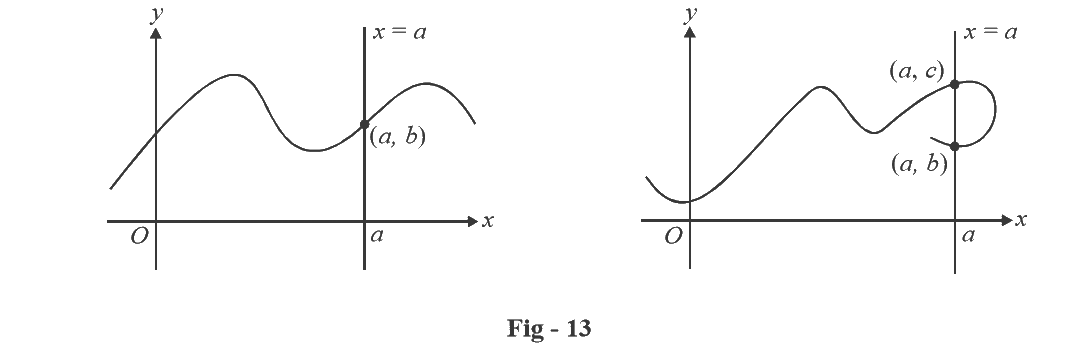For example, the parabola $$x = {y^2} - 2$$ shown in Figure 14(a) is not the graph of a function of $$x$$ because, as you can see, there are vertical lines that intersect the parabola twice. The parabola, however, does contain the graphs of two functions of $$x$$. Notice that the equation $$x = {y^2} - 2$$ implies $${y^2} = x + 2$$, so $$y = \pm \sqrt {x + 2}$$. Thus, the upper and lower halves of the parabola are the graphs of the functions $$f\left( x \right) = \sqrt {x + 2}$$ and $$g\left( x \right) = - \sqrt {x + 2}$$.

[See figures 14(b) and (c)]. We observe that if we reverse the roles of x and y, then the equation $$x = h\left( y \right) = {y^2} - 2$$ does define x as a function of y (with y as the independent variable and x as the dependent variable) and the parabola now appears as the graph of the function $$h$$.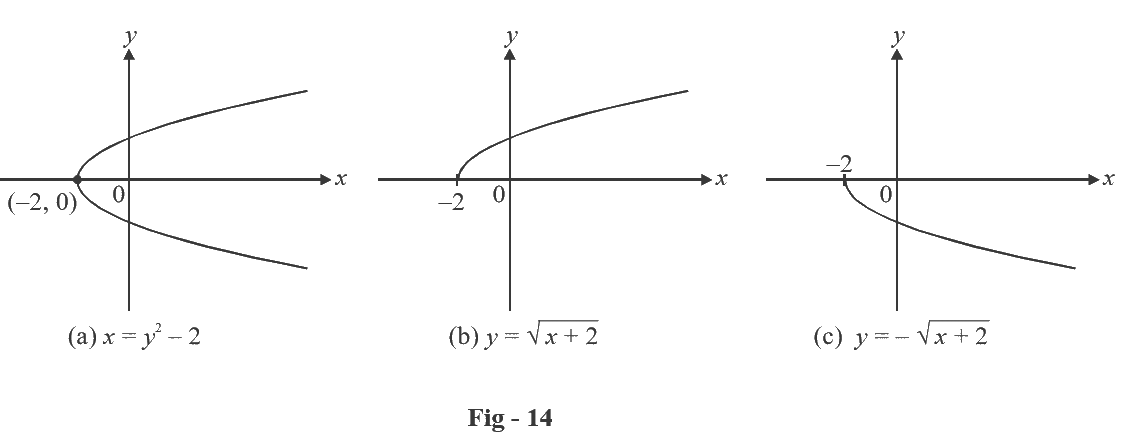The functions in the following examples are defined by different formulas in different parts of their domains. Such functions are called piecewise defined functions.

Example- 5

A function $$f$$ is defined by

f\left( x \right) = \left\{ \begin{align}&1 - x & {\rm{if \;\;}}x \le 1\\&{x^2} & {\rm{if \;\;}}x > 1\end{align} \right.

Evaluate $$f\left( 0 \right),\;f\left( 1 \right),\;{\rm{and }}f\left( 2 \right)$$ and sketch the graph.

Solution: Remember that a function is a rule. For this particular function the rule is the following. First look at the value of the input x. If it happens that $$x \le 1$$, then the value of $$f\left( x \right)$$ is $$1 - x.$$ On the other hand, if $$x > 1$$, then the value of $$f\left( x \right)$$ is $${x^2}$$.

Since $$0 \le 1,$$ we have $$f\left( 0 \right) = 1 - 0 = 1$$

Since $$1 \le 1,$$ we have $$f\left( 1 \right) = 1 - 1 = 0$$

Since $$2 > 1,$$ we have $$f\left( 2 \right) = {2^2} = 4$$

How do we draw the graph of $$f\,?$$ We observe that if $$x \le 1$$, then $$f\left( x \right) = 1 - x,$$ so the part of the graph of f that lies to the left of the vertical line $$x = 1$$ must coincide with the line $$y = 1 - x,$$ which has slope –1 and y – intercept 1. If $$x > 1,$$ then $$f\left( x \right) = {x^2}$$, so the part of the graph of f that lies to the right of the line $$x = 1$$ must coincide with the graph of $$y = {x^2}$$, which is a parabola. This enables us to sketch the graph in the figure. The solid dot indicates that the point (1, 0) is included on the graph; the open dot indicates that the point (1, 1) is excluded from the graph.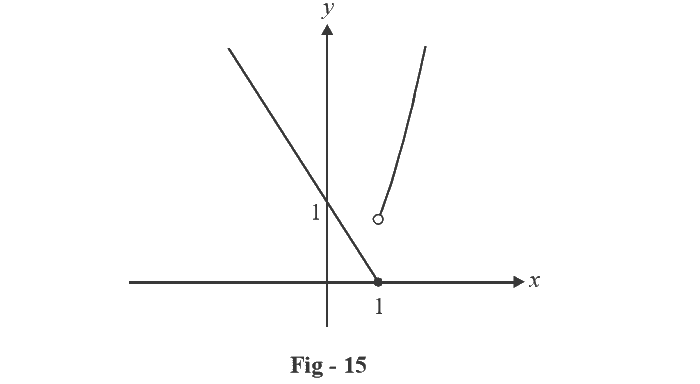The next example of a piecewise defined function is the absolute value function. Recall that the absolute value of a number a, denoted by |a|, is the distance from a to 0 on the real number line. Distances are positive or 0, so we have

$$\left| a \right| \ge 0$$ for every number a

For example,

\begin{align}&\left| 3 \right| = 3\;\;\;\;\;\left| { - 3} \right| = 3\;\;\;\;\;\left| 0 \right| = 0\;\;\;\;\;\left| {\sqrt 2 - 1} \right| = \sqrt 2 - 1 \;\;\;\;\;\left| {3 - \pi } \right| = \pi - 3\end{align}

In general, we have

$$\fbox{{\left| a \right| = a\quad {\rm{\;if}}\;a \ge 0\\\left| a \right| = - a\quad {\rm{\;if \;}}a < 0}}$$

(Remember that if $$a$$ is negative, then $$–a$$ is positive).

Example- 6

Sketch the graph of the absolute value function $$f\left( x \right) = \left| x \right|$$

Solution: From the preceding discussion we know that

\left| x \right| = \left\{ \begin{align}&\quad x & {\rm{if\;}}\;x \ge 0\\&- x & {\rm{if \;\;}}x < 0\end{align} \right.

Using the same method as in the previous example, we see that the graph of f coincides with the line $$y = x$$ to the right of the y -axis and coincides with the line $$y = - x$$ to the left of the y-axis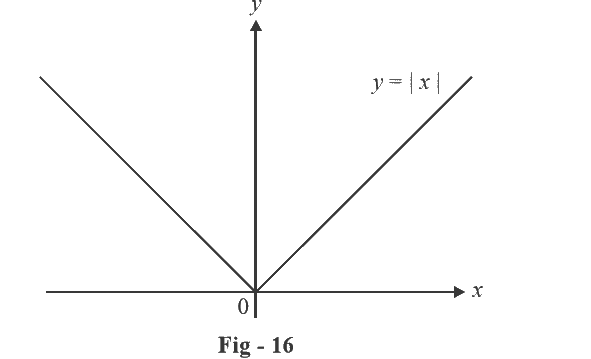Example- 7

Find a formula for the function f graphed in Figure - 17.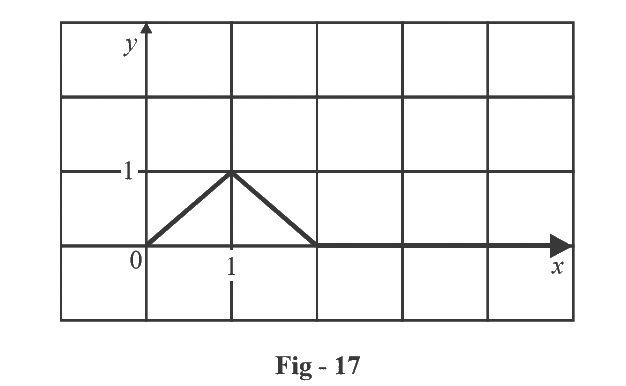Solution: The line through (0, 0) and (1, 1) has slope $$m = 1$$ and y-intercept $$b = 0$$, so its equation is $$y = x$$. Thus for the part of the graph f that joins (0, 0) to (1, 1), we have

$f\left( x \right) = x\quad {\rm{if\;\; 0}} \le x \le {\rm{1}}$

The line through (1, 1) and (2, 0) has slope $$m = - 1$$, so its point - slope form is

$y - 0 = \left( { - 1} \right)\left( {x - 2} \right)\quad {\rm{or \;\;\;}} y = 2 - x$

So we have

$\,f\left( x \right) = 2 - x\quad {\rm{if \;\;}}1 < x \le 2$

We also see that the graph of f coincides with the x-axis for $$x > 2$$. Putting this information together, we have the following three - piece formula for $$f$$ :

f\left( x \right) = \left\{ \begin{align}&x & & {\rm{if\quad }}0 \le x \le 1\\&2 - x & & {\rm{if \quad}}1 < x \le 2\\&0 & & {\rm{if \quad }}x > 2\end{align} \right.

## TRY YOURSELF - I

Q.1:  An equilateral triangle is inscribed in circle of radius r. Find the area A of the triangle as a function of r.

Q.2:  Let $$y = f\left( x \right)$$be such that \begin{align}&\frac{{{x^2}}}{{{a^2}}} + \frac{{{y^2}}}{{{b^2}}} = 1.\end{align}Is $$f\left( x \right)$$ a function?

Q.3:  Find a formula for the function graphed below: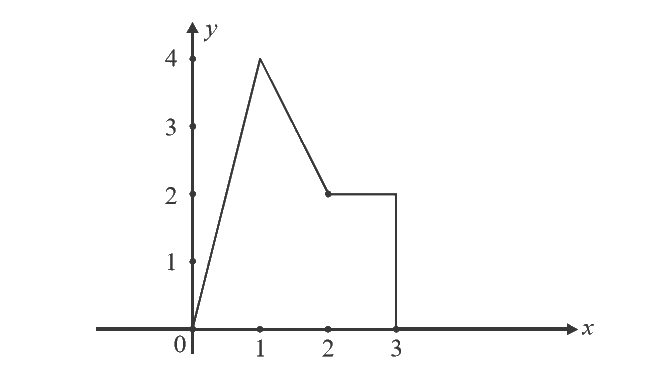Q.4:  A projectile is thrown with an initial velocity u at an angle $$\theta$$ to the horizontal. Let $$(x,y)$$ be its position at some given time t.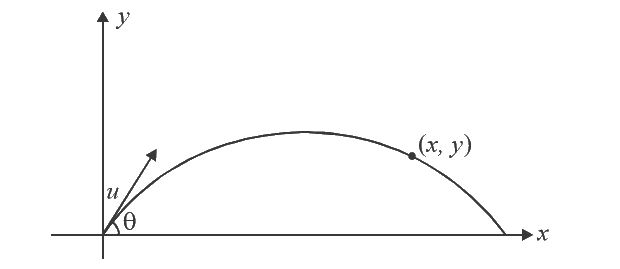Find

(a) x as a function of t

(b) y as a function of t

(c) y as a function of x

(d) the distance r of the projectile from the origin, as a function of t.